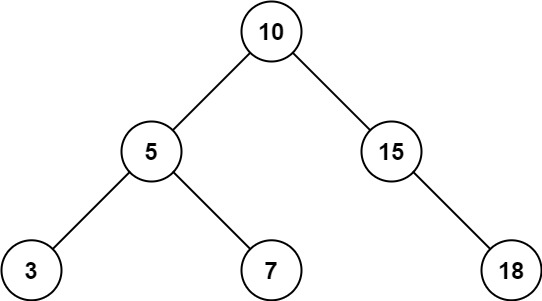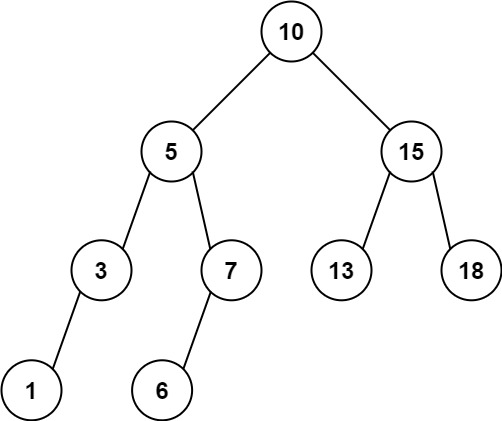# 938. Range Sum of BST

Medium
Given the `root` node of a binary search tree and two integers `low` and `high`, return the sum of values of all nodes with a value in the inclusive range `[low, high]`.
Example 1:Input: root = [10,5,15,3,7,null,18], low = 7, high = 15
Output: 32
Explanation: Nodes 7, 10, and 15 are in the range [7, 15]. 7 + 10 + 15 = 32.
Example 2:Input: root = [10,5,15,3,7,13,18,1,null,6], low = 6, high = 10
Output: 23
Explanation: Nodes 6, 7, and 10 are in the range [6, 10]. 6 + 7 + 10 = 23.
Constraints:
• The number of nodes in the tree is in the range `[1, 2 * 104]`.
• `1 <= Node.val <= 105`
• `1 <= low <= high <= 105`
• All `Node.val` are unique.

### 解題

/**
* Definition for a binary tree node.
* type TreeNode struct {
* Val int
* Left *TreeNode
* Right *TreeNode
* }
*/
func rangeSumBST(root *TreeNode, low int, high int) int {
ans := 0
var helper func(*TreeNode)
helper = func(root *TreeNode) {
if root == nil {
return
}
if root.Val <= high && root.Val >= low {
ans += root.Val
}
helper(root.Left)
helper(root.Right)
}
helper(root)
return ans
}